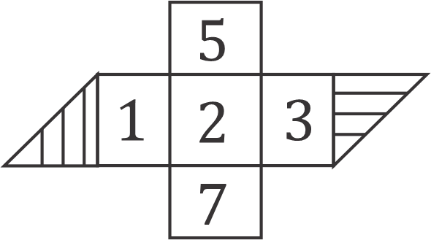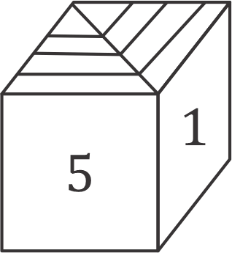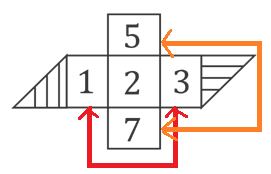Question of The Day16-07-2020

When the given 2D figure is folded to form the 3D cube, then which of the following option correctly depicts one of the positions of 3D cube?Correct Answer : d )Explanation :

These types of questions can be solved using the logic given below:

• In the 2D question figure if there are two faces or squares in a straight line, then the two faces will never be opposite to each other in the final 3D cube.
• In the 2D question figure if there are more than 2 faces or squares in a straight line, then the faces or squares at a gap of one block in the straight line will always be opposite to each other.

Thus, considering the given 2D figureWe can say that, in the above figure

‘7’ will be opposite to ‘5’

‘1’ will be opposite to ‘3’

And, the remaining ‘shaded part’ will be opposite to ‘2’

As, in option (a), ‘1’ and ‘3’ are on the adjacent faces which cannot be possible as both the numbers can be only on the opposite faces. Thus, option (a) is incorrect.

Similarly, in option (b): ‘7’ and ‘5' are on the adjacent faces which cannot be possible as both the numbers can be only on the opposite faces. Thus, option (b) is incorrect.

Now, considering option (c): ‘shaded part’ and ‘2’ are on the adjacent faces which cannot be possible as both the numbers can be only on the opposite faces. Thus, option (c) is also incorrect.

Thus, we can say that option (d) is the correct answer as it does not violate any condition.

Hence, (d) is the correct answer.

Such type of question is asked in various government exams like SSC CGL, SSC MTS, SSC CPO, SSC CHSL, RRB JE, RRB NTPC, RRB GROUP D, UPSC CDS, UPSC NDA etc.

Read Daily Current Affairs, Banking Awareness, Hindi Current Affairs, Word of the Day, and attempt free mock tests at PendulumEdu and boost your preparation for the actual exam.0